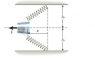# Physics- mechanical energy

J.live

## Homework Statement

A horizontal slingshot consists of two light, identical springs (with spring constants of 74 N/m) and a light cup that holds a 1-kg stone. Each spring has an equilibrium length l0 = 49 cm. When the springs are in equilibrium, they line up vertically. Suppose that the cup containing the mass is pulled to x = 0.6 m to the left of the vertical and then released.

## The Attempt at a Solution

ME= PE +KE

Initially PE = 1/2kx^2 KE= 0 --> ME = 1/2kx^2 +0 ---> 1/2(74)(.6)^2 +0 ?

Idk how to find the velocity. I am guessing we'll just use ME= 0+1/2mv^2 ?

Or, do I have to incorporate the equilibrium length into the equation somehow?

Any help will be appreciated.

#### Attachments

•Screen shot 2010-10-04 at 3.19.41 PM.png
5.3 KB · Views: 416
Last edited:

J.live
anyone ?

Mentor
Or, do I have to incorporate the equilibrium length into the equation somehow?
Definitely. Figure out how much the springs are stretched from their equilibrium lengths when the cup is moved. (It's not simply 0.6 m.) Then your energy approach will work.

J.live
How do I calculate that ?:/

Edit: Pythagorean Theorem ?

Mentor
How do I calculate that ?:/
How long are the stretched springs? How does that compare with their unstretched length?

Yes, Pythagorean theorem!

J.live
This is what I did

C^2 = .6^2 + .49 ^2 = .77 m

Total ME = 1/2(74)(.77)^2 +0 = 21. 97 J ?

That seems off. Am I suppose to subtract and add the x and y components before applying the Pythagorean Theorem ?

If so then isn't y = 0 since they are in opposite directions? x = .8 +.8 = 1.6 ?

I am still kind of confused.

Edit- I just tried it that makes no sense whatsoever. :/ What am i doing wrong ?

Last edited:
J.live
When I subtract the values I am getting the right answer. C^2= .64^2 - .48^2 ?

Mentor
This is what I did

C^2 = .6^2 + .49 ^2 = .77 m
You correctly found the stretched length of the springs. So how much did they stretch compared to their unstretched length? That elongation will tell you the amount of stored elastic potential energy.

J.live
I don't understand which value you are referring to exactly as unstretched length?

.49 m?

Last edited:
Mentor
I don't understand which value you are referring to exactly as unstretched length?

.49 m?
Yes, what they are calling the equilibrium length or l0. To find the energy stored in the stretched springs, you need to know how far they are stretched from their equilibrium positions.

J.live
So, will it be .77 -.49 = .28 ?

Mentor
So, will it be .77 -.49 = .28 ?
Good.

J.live
Now I plug it in the equation

ME = 1/2kx^2 +0 --> 1/2 74 (.28)^2= 2.9 J ?

Mentor
Now I plug it in the equation

ME = 1/2kx^2 +0 --> 1/2 74 (.28)^2= 2.9 J ?
That's the energy stored in each spring.

J.live
ME= 2*(1/2 ) 74 (.28)^2 = 5.99 j

Last edited: